# Circle sector

The circular sector with a central angle 160° has an area 452 cm2. Calculate its radius r.

r =  17.9923 cm

### Step-by-step explanation: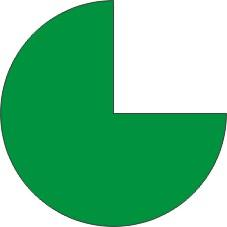Did you find an error or inaccuracy? Feel free to write us. Thank you!#### You need to know the following knowledge to solve this word math problem:

We encourage you to watch this tutorial video on this math problem:

## Related math problems and questions:

• Cone A2VThe surface of the cone in the plane is a circular arc with central angle of 126° and area 415 cm2. Calculate the volume of a cone.
• SectorThe perimeter of a circular sector with an angle 1.8 rad is 64 cm. Determine the radius of the circle from which the sector comes.
• Arc-sectorarc length = 17 cm area of sector = 55 cm2 arc angle = ? the radius of the sector = ?
• Four circles1) Calculate the circle radius if its area is 400 cm square 2) Calculate the radius of the circle whose circumference is 400 cm. 3) Calculate circle circumference if its area is 400 cm square 4) Calculate the circle's area if perimeter 400 cm.
• Circle arcCalculate the circular arc area in m2 where the diameter is 263 dm, and a central angle is 40°. Result round to three decimal places.
• Circular sector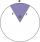I have a circular sector with a length 15 cm with an unknown central angle. It is inscribed by a circle with radius 5 cm. What is the central angle alpha in the circular sector?
• Chord MNChord MN of circle has distance from the center circle S 120 cm. Angle MSN is 64°. Determine the radius of the circle.
• Circular segmentCalculate the area S of the circular segment and the length of the circular arc l. The height of the circular segment is 2 cm and the angle α = 60°. Help formula: S = 1/2 r2. (Β-sinβ)
• Quarter circleWhat is the radius of a circle inscribed in the quarter circle with a radius of 100 cm?
• A spherical segment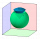A spherical section whose axial section has an angle of j = 120° in the center of the sphere is part of a sphere with a radius r = 10 cm. Calculate the cut surface.
• Triangle SASCalculate the triangle area and perimeter, if the two sides are 51 cm and 110 cm long and angle them clamped is 130 °.
• Disc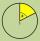The circumference of the disk is 78.5 cm. What is the circumference of the circular arc of 32° on the disc?
• Diamond and anglesFind the area of a diamond with a side of 5 cm if you know that the internal angles in the diamond are 60° and 120°.
• NonagonCalculate the area and perimeter of a regular nonagon if its radius of the inscribed circle is r = 10cm
• Quarter of a circleCalculate the circumference of a quarter circle if its content is S = 314 cm2.
• Cuboid - volume and areasThe cuboid has a volume of 250 cm3, a surface of 250 cm2, and one side 5 cm long. How do I calculate the remaining sides?
• Arc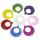What area of a circle occupied the flowers planted in the arc of a circle with radius 3 m with central angle 45°?